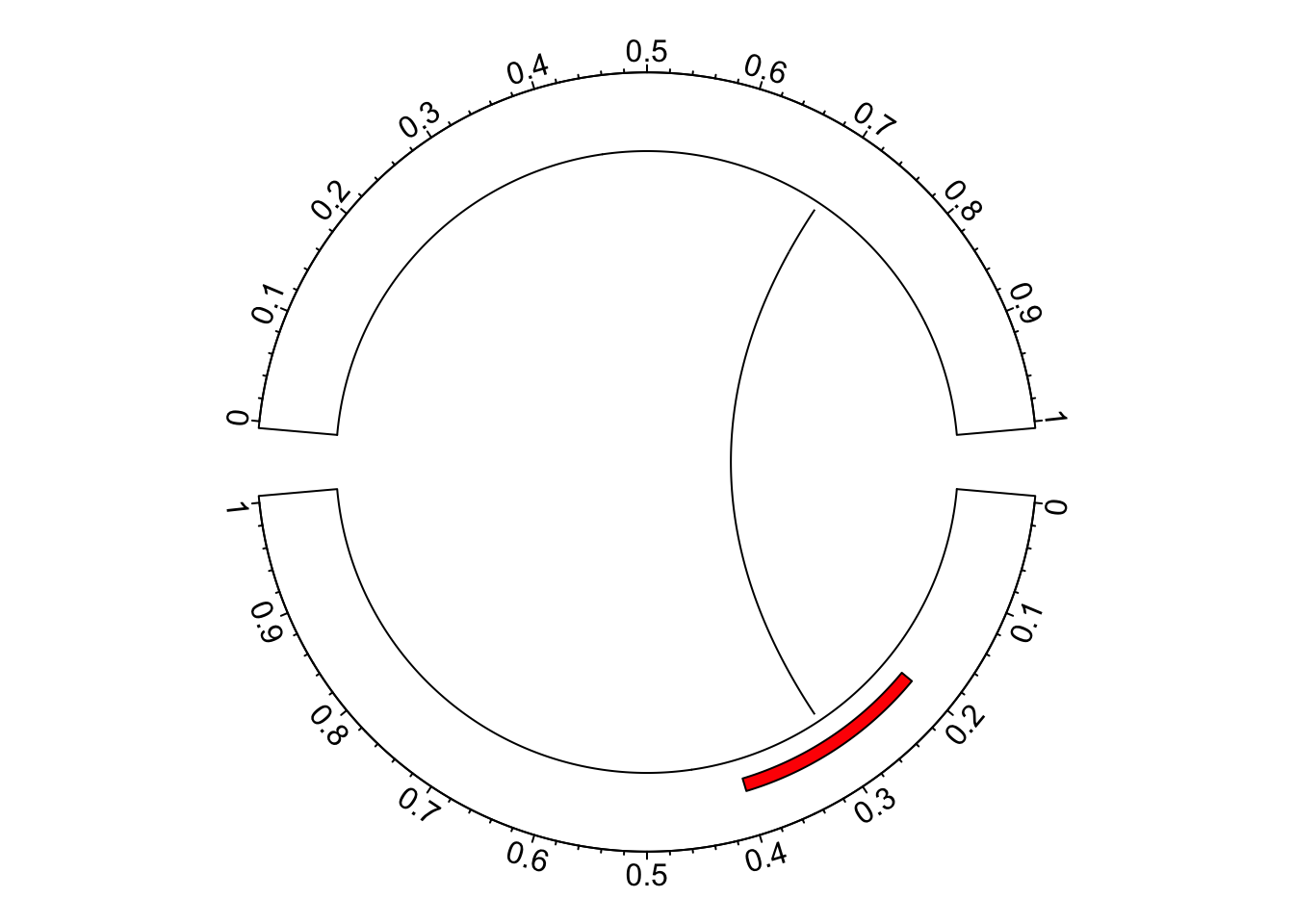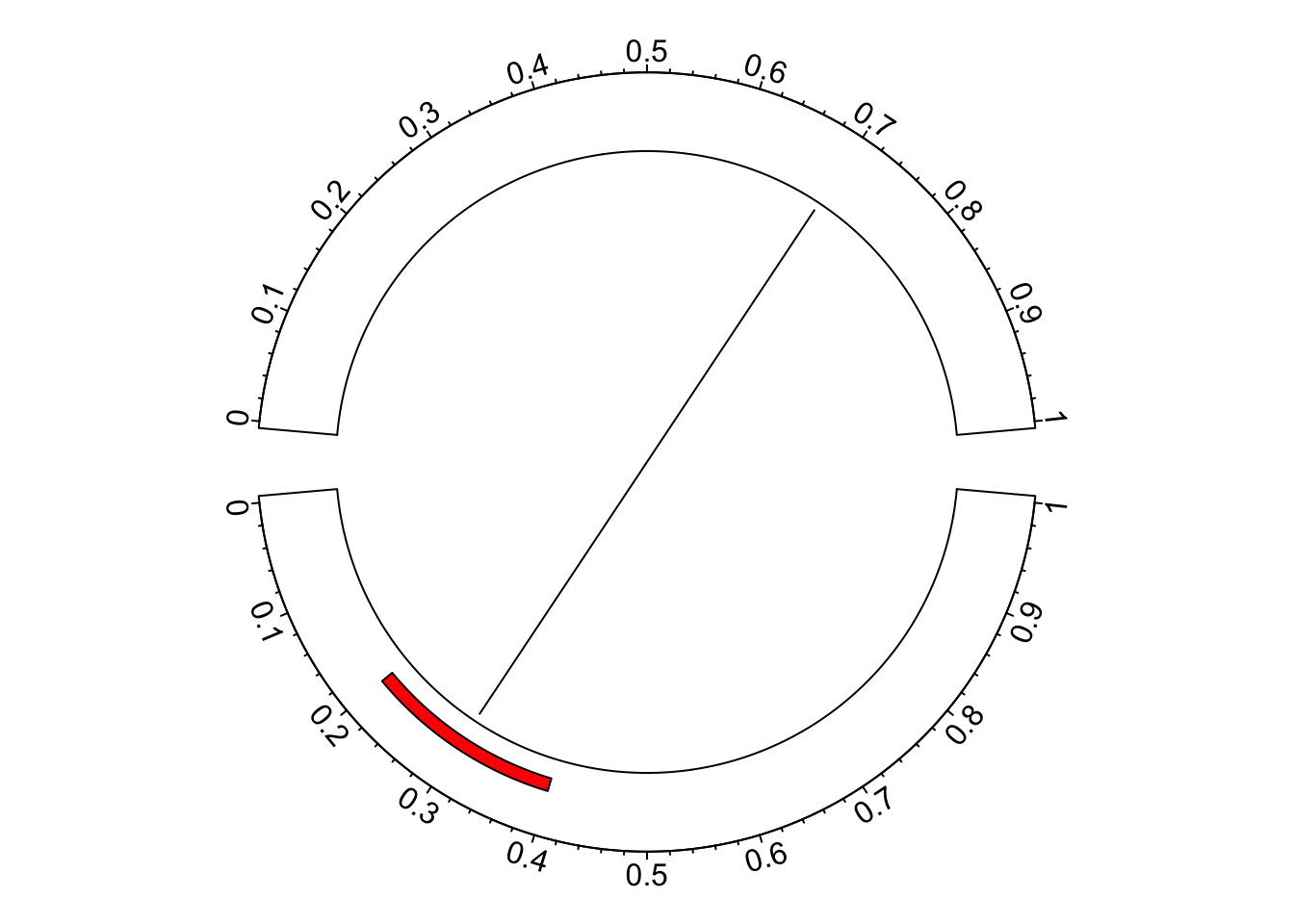# Reverse x-axis in the circular layout

In circlize package, x-axis in always clockwise in each sector, no matter it is on the top of the circle or at the bottom. However, you can manually reverse the original positions on x-axis to pretend you have a reversed x-axis. Take following example:

``library(circlize)``
``````## ========================================
## circlize version 0.4.9
## CRAN page: https://cran.r-project.org/package=circlize
## Github page: https://github.com/jokergoo/circlize
## Documentation: https://jokergoo.github.io/circlize_book/book/
##
## If you use it in published research, please cite:
## Gu, Z. circlize implements and enhances circular visualization
##   in R. Bioinformatics 2014.
##
## This message can be suppressed by:
##   suppressPackageStartupMessages(library(circlize))
## ========================================``````
``````circos.par(gap.degree = c(10, 10), start.degree = -5)
circos.initialize(factors = c("a", "b"), xlim = c(0, 1))
circos.trackPlotRegion(ylim = c(0, 1), panel.fun = function(x, y) {
circos.axis(major.at = seq(0, 1, by = 0.1))
})
circos.rect(0.2, 0.2, 0.4, 0.4, col = "red", sector.index = "a")``circos.clear()``

In the bottom sector, the order of x-axis seems not very convinient to read. Actually we can make some transformation on the original coordinates on x-axis. In following we defined `reverse_xaxis()` which flips the x-axis.

``````reverse_xaxis = function(x, xlim = get.cell.meta.data("xlim")) {
(xlim - xlim) - (x - xlim) + xlim
}
reverse_xaxis(seq(0, 1, by = 0.1), xlim = c(0, 1))``````
``##   1.0 0.9 0.8 0.7 0.6 0.5 0.4 0.3 0.2 0.1 0.0``

In the arguments of `reverse_xaxis()`, the default value for `xlim` is `get.cell.meta.data("xlim")` so that it can automatically get the ranges on x-axis if `reverse_xaxis()` is called inside `panel.fun()`. If it is called somewhere else, `xlim` should be defined explicitly.

Now we apply `reverse_xaxis()` to all the coordinates in the sectors we want to flip.

``````circos.par(gap.degree = c(10, 10), start.degree = -5)
circos.initialize(factors = c("a", "b"), xlim = c(0, 1))
circos.trackPlotRegion(ylim = c(0, 1), panel.fun = function(x, y) {
sector.index = get.cell.meta.data("sector.index")
at = seq(0, 1, by = 0.1)
if(sector.index == "a") {
circos.axis(major.at = at, labels = reverse_xaxis(at))
circos.rect(reverse_xaxis(0.2), 0.2, reverse_xaxis(0.4), 0.4,
col = "red", sector.index = "a")
} else {
circos.axis(major.at = at)
}
})
xlim = get.cell.meta.data("xlim", sector.index = "a")``circos.clear()``